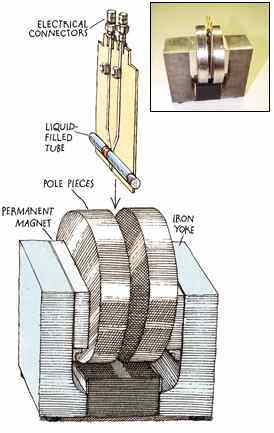Tech SectionTechnology -> Quantum Computers

Quantum Computers
Introduction

I am sure most of you would like to be able to break the encryption code of major banks in a few seconds so that you can empty the bank vault. Until now, this was just a dream but not anymore. With recent development in a new field of computing researchers are claiming that it is possible to break the RSA 512 codes in milliseconds.

So, what is this Quantum computing and what makes it so special? Quantum computing is a new field with a great advantage over conventional computing. The advantage of the quantum computer arises from the way it stores data. The conventional computer stores data in the form of bits with 8 bits making a byte. The bit can have a value of either '0' or '1' represented by the presence or absence of an electric charge. Thus the bit is the fundamental unit of information in a conventional computer. The fundamental unit of a quantum computer is called a quantum bit or a qubit and is represented by an atom in one of two different states, which can also be denoted as 0 or 1. But unlike the normal bit the qubit can exist simultaneously as 0 and 1 with the probability for each state given by a numerical coefficient. Describing a two-qubit quantum computer thus requires four coefficients. In general, n qubits demand 2^n numbers, which rapidly becomes a sizable set for larger values of n.

A quantum computer promises to be immensely powerful because it can be in multiple states at once -- a phenomenon called superposition and because it can act on all its possible states simultaneously. Thus, a quantum computer could naturally perform big operations in parallel, using only a single processing unit.

In 1994 Peter W. Shor of AT&T deduced how to take advantage of entanglement and superposition to find the prime factors of an integer. He found that a quantum computer could, in principle, accomplish this task much faster than the best classical calculator ever could. He then proceeded to write an algorithm called Shor's algorithm which prompted computer scientists to begin learning about quantum mechanics.

The scientists working on building a quantum computer soon realized that building a quantum computer would be very difficult. The main problem being that almost any interaction with a quantum system has with its environment -- say, an atom colliding with another atom or a stray photon constitutes a measurement. The superposition of quantum mechanical states then collapses into a single very definite state :- the one that is detected by an observer. This phenomenon, known as decoherence, makes further quantum calculation impossible. Thus, the inner workings of a quantum computer must somehow be separated from its surroundings to maintain coherence. But they must also be accessible so that calculations can be loaded, executed and read out. This problem baffled the scientists and may attempts were made to solve this problem by scientists like Christopher R. Monroe and David J. Wineland of the National Institute of Standards and Technology and by H. Jeff Kimble of the California Institute of Technology. But even their heroic experimental efforts have demonstrated only rudimentary quantum operations, because these novel devices involve only a few bits and because they lose coherence very quickly.

So how then can a quantum-mechanical computer ever be exploited if it needs to be so well isolated from its surroundings? It turns out that filling a test tube with a liquid made up of appropriate molecules - that is, using a huge number of individual quantum computers instead of just one -- neatly addresses the problem of decoherence. By representing each qubit with a vast collection of molecules, one can afford to let measurements interact with a few of them. Nuclear magnetic resonance operates on quantum particles in the atomic nuclei within the molecules of the fluid. Particles with "spin" act like tiny bar magnets and will line up with an externally applied magnetic field. Two alternative alignments (parallel or antiparallel to the external field) correspond to two quantum states with different energies, which naturally constitute a qubit. It allows a logic "gate," the basic unit of a computation, to be readily constructed using two nuclear spins. This was the basis of the first Quantum computer built in 1996. The first computation accomplished that exercised the unique abilities of quantum-mechanical computing followed an ingenious search algorithm devised by Lov K. Grover of Bell Laboratories.

So quantum computers might be built. But how fast would they be? The effective cycle time of a quantum computer is determined by the slowest rate at which the spins flip around. This rate is typically ranges from hundreds of cycles a second to a few cycles a second. Although running only a handful of clock cycles each second might seem awfully sluggish compared with the megahertz speed of conventional computers, a quantum computer with enough qubits would achieve such massive quantum parallelism that it would still factor a 400-digit number in about a year.A desktop Quantum Computer model

This makes one wonder how long it would take for a desktop model of a quantum computer to be common. At present the wait for a desktop quantum computer is long. The diagram above shows the essential elements of a tabletop quantum computer as being assembled by researchers.

The quantum computer may be a 100 years off or may be invented in the next decade but we can be sure that when they are invented they will change the face of computing forever.